How Cheenta works to ensure student success?
Explore the Back-Story

# Test of Mathematics Solution Subjective 61 - Symmetric PolynomialThis is a Test of Mathematics Solution Subjective 61 (from ISI Entrance). The book, Test of Mathematics at 10+2 Level is Published by East West Press. This problem book is indispensable for the preparation of I.S.I. B.Stat and B.Math Entrance.

Also visit: I.S.I. & C.M.I. Entrance Course of Cheenta

## Problem

Solve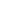- 25x + 12 +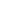+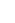= 0.

## Solution– 25x + 12 ++= 0
= 6(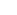+) – 25(x -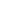) +12 = 0
= 6 (x –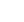– 25 (x –) + 24 = 0
= (x –) =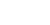=orIf (x –) =Or– 3x – 2 = 0
Or x = 2 , -If (x –) =Or– 1 =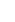Or– 8x – 3 = 0
Or x = 3, -1/3
X = 2,-,3,-This is a Test of Mathematics Solution Subjective 61 (from ISI Entrance). The book, Test of Mathematics at 10+2 Level is Published by East West Press. This problem book is indispensable for the preparation of I.S.I. B.Stat and B.Math Entrance.

Also visit: I.S.I. & C.M.I. Entrance Course of Cheenta

## Problem

Solve- 25x + 12 ++= 0.

## Solution– 25x + 12 ++= 0
= 6(+) – 25(x -) +12 = 0
= 6 (x –– 25 (x –) + 24 = 0
= (x –) ==orIf (x –) =Or– 3x – 2 = 0
Or x = 2 , -If (x –) =Or– 1 =Or– 8x – 3 = 0
Or x = 3, -1/3
X = 2,-,3,-### Knowledge Partner